# Batch Process Implementation in Kettle

#### Batch Process Implementation in Kettle (Pentaho Data Integration)

In order to implement the batch process we needs to have the looping logic. But I did not find any component or suitable method in kettle to create those loops.

Make data easy with Helical Insight.
Helical Insight is the world’s best open source business intelligence tool.

Get your 30 Days Trail Version

So I have created a way to resolve that problem in one of my project and updated the same here.

Below is the step by step process:

1.Get Batch Details:

Create a new transformation: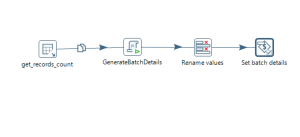Create a separate transformation and name it as get_batch_details.

Fetch min, max and batch size details from the source system and calculate total number of batches count using java script step.

Calculate total batch number count: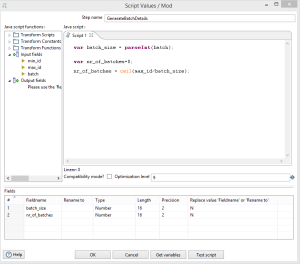Set each variable scope to valid thorough out the root job using set variable step like below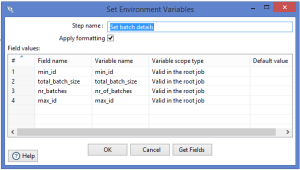2.Create a main job:

Create a main job with the following steps.

```        a. configure get_batch_details transformation.
b. configure for each batch evaluation step
c. configure your actual transformation with transformation executor step
d. configure batch number iteration transformation
```

Main job will looks like below.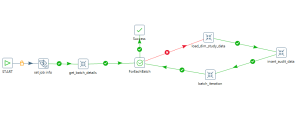3.Evaluate and iterate through each batch number in main job:

Evaluation:

In this step we have to evaluate each batch number with the total number of batches like  below.

This process will continue until the condition set to true.

For each step configuration: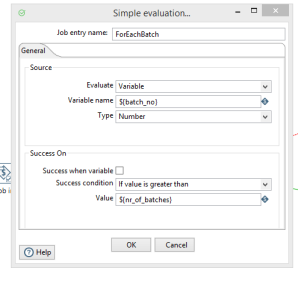In main job create a variable with the name as batch_no and assign some default value to it.

And make use same variable in evaluation step like above and make sure to have the success condition as If value is greater than and the value as nr_of_batches. (This value we are getting from get_batch_details transformation)

So for the first time batch_no = 1, then it will compare with nr_of_batches each time, if the condition is false then your actual job will execute other wise it will exit from the loop.

Make data easy with Helical Insight.
Helical Insight is the world’s best open source business intelligence tool.

Claim Your 30 Days Free Trail

Now the next step is to increment the batch_no, so we have to create a batch_iteration transformation like below and configure get and set variable steps accordingly.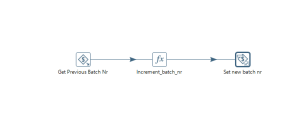Increment batch number step:

Use formula step to implement this logic and configure the values as shown like below.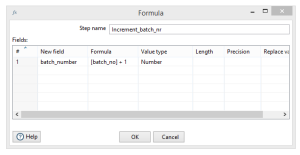We have configured everything properly but the main thing is to how to make use batch info and create start and end flags in our actual transformation.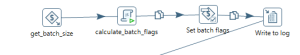Calculate batch flags using java script step like below.So use start flag and end flag details wherever required in the job.

For example:

step 1: calculate total number of batches

```      max value = 12000,

batch_size = 4000,

nr_of_batches = ceil (12000)/4000 = 3```

So in this process it will create 3 different batches with batch size as 4000 each time.

step 2: evaluate batch number and total number of batches in main job.

```      nr_of_batches = 3;

batch_no = 1 (default);

If (batch_no > nr_of_batches)

then exit

else go to actual job.```

So here, 1> 3 which is false then it will go to actual job.

step 3: calculate batch flags in actual transformation.

```    var start_flag=0;

var end_flag=0;

start_flag=(batch_no - 1)*batch_size + 1;

end_flag= (batch_no*batch_size);

start_flag = (1-1)*4000+1;

end_flag = (1*4000);

Now start_flag=1 and end_flag = 4000;

```

So we can use above flag values in our query to fetch data from the sources system using any table input step.

Step 4: Increment batch number

`      batch_no = batch_no + 1;`

So now the batch_no = 2 and the same process will continue until the condition set to true.Best Open Source Business Intelligence Software Helical Insight is Here